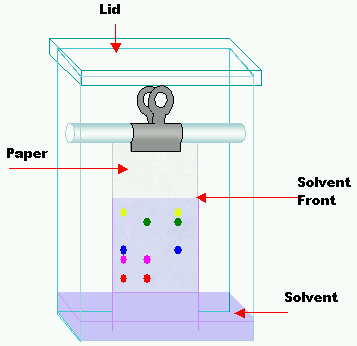## Molar Solubility and Relative Solubility

#### Learning Objective

• Calculate the molar solubility of a compound in water and the relative molar solubility of compounds

#### Key Points

• A substance’s solubility product (Ksp) is the ratio of concentrations at equilibrium.
• The molar solubility of a compound can be calculated directly from its solubility product.
• Even if the solubility products of two compounds are similar, their molar solubilities can be very different.
• Scientists take advantage of the relative solubilities of compounds to separate or identify them.

#### Terms

• literA non-SI metric system unit of volume equal to 1 cubic decimeter (dm3), 1,000 cubic centimeters (cm3) or 1/1,000 cubic meter (m3)
• molar solubilityThe number of moles of a substance (the solute) that can be dissolved per liter of solution before the solution becomes saturated.
• saturatedContaining all the solute that can normally be dissolved at a given temperature.
• saturated solutionOne in which the solvent can dissolve no more of a specific solute at a particular temperature.

## Molar Solubility

A substance’s solubility product (Ksp) is the ratio of concentrations at equilibrium. Molar solubility, which is directly related to the solubility product, is the number of moles of the solute that can be dissolved per liter of solution before the solution becomes saturated. Once a solution is saturated, any additional solute precipitates out of the solution. The units are molarity (M), or mole liter-1 (mol/L).

## Calculating Molar Solubility

The relation between the molar solubility and the solubility product means that one can be used to find the other.

Example 1:

The Ksp for AgI is 8.5 x 10-17 at 25 °C. What is the molar solubility? (Let s = the solubility of the compound in water, usually defined as x in an ICE table.)

Solution:

The balanced equation for the reaction is:

$AgI (s) \leftrightarrow Ag^+ (aq) + I^- (aq)$

The formula for Ksp is:

Ksp = [Ag+][I]

Ksp = s2 = 8.5 x 10-17

where s is the concentration of each ion at equilibrium. Now, solve for s:

s2 = 8.5 x 10-17

s = $\sqrt {8.5 \times 10^{-17} }$

s = 9.0 x 10-9 mol/L

The molar solubility of AgI is 9.0 x 10-9 mol/L.

Example 2:

The solubility products for cadmium carbonate (CdCO3) and silver carbonate (Ag2CO3) are almost exactly the same. Compare their molar solubilities in water at 25 °C.

Solution:

$CdCO_3 (s) \leftrightarrow Cd^{2+} (aq) + CO_3\ ^{2+} (aq)$

Ksp = [Cd2+][CO32-]

s2 = 5.2 x 10-12

s = 2.3 x 10-6 mol/L

For silver carbonate, the expression is slightly different. Since each mole of salt produces two moles of Ag+ ions, the value of Ag+ is 2s:

$Ag_2CO_3 (s) \leftrightarrow 2Ag^+ (aq) + CO_3^{2-} (aq)$

Ksp = [Ag+]2[CO32-]

(2s)2s = 8.2 x 10-12

4s3 = 8.2 x 10-12

s = 1.3 x 10-4 mol/L

Although cadmium carbonate and silver carbonate have nearly the same solubility products, their solubilities in mol/L differ by a factor of 100. The solubility of silver carbonate is sensitive to the square of the metal-ion concentration because two silver ions per carbonate ion are necessary to build the solid crystal. Therefore, the form of the solubility product expression is different.

## Relative Solubility

If compounds have different solubilities, or relative solubilities, they can be separated. For example, in the process of extraction, scientists take something dissolved in one liquid and force it to become dissolved in another liquid. Caffeine must be extracted from coffee beans in order to be used in beverages such as soda. Typically, the caffeine is dissolved in carbon dioxide that has been heated to over 300 K and pressurized to 73 atm, making it a liquid. Then, the temperature is lowered (lowering the solubility of the caffeine in carbon dioxide), and water is added. The system is allowed to reach equilibrium. Since caffeine is more soluble in water than it is in carbon dioxide, the majority of it goes into the water.

A technique called paper chromatography also takes advantage of the relative solubilities of compounds. In paper chromatography, a small amount of the mixture is placed onto the paper approximately 1 cm from the edge. Then, the paper is suspended in a small quantity of the solvent in a closed container. As the solvent rises up the paper, the components in the mixture separate based on relative solubility. As the solvent nears the top, a mark is made to record the level, and the paper is removed and dried. Some components are colored and can be seen with the naked eye, but some need to be stained or irradiated with an ultraviolet lamp. The solute will always move at the same fraction of the distance of the solvent as long as temperature is held constant. The distance that the solute travels in a particular solvent can be used to identify the compound.Schematic of paper chromatographyPaper chromatography is an analytical chemistry technique for separating and identifying mixtures. Samples are spotted on the bottom of the paper. The end of the paper is placed in solvent. The solvent travels up the paper through capillary action. Mixtures are separated by their relative solubility in the solvent.